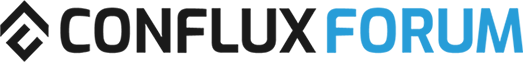# Conflux DAO# 10 The multiplier of the staking interest rate of PoS from the basic rate of 4% / PoS 质押利率相比 现有4% 基础质押利率的加成系数

This vote is to determine the multiplier of the staking interest rate of PoS from the basic rate of 4%, assuming PoS Finality is introduced with the next network upgrade. (Set x = circulating supply of CFX / total amount of CFX staked)

If the multiplier is √x and the total amount of staked CFX on the PoS chain is relatively low, the staked votes will receive more interest. The multiplier of √x will encourage more people to participate it and guarantees the total inflation rate of CFX token to remain below 4%.

## Voting Options:

A. √x (e.g.， when x = 4, the interest rate is 8; when x = 9, the interest rate is 12%.; and so on)
B. 1 (the interest rate of PoS remains as 4%)

## 选项/Option:

A.√x （当质押量为流通量的 1/4 时，利率为 8%；当质押量为流通量 1/9 时，利率为 12%；以此类推）
B.1 （利率始终为 4%）